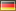## Testing the Significance of Correlations#### 1. Comparison of correlations from independent samples

Correlations, which have been retrieved from different samples can be tested against each other. Example: Imagine, you want to test, if men increase their income considerably faster than women. You could f. e. collect the data on age and income from 1 200 men and 980 women. The correlation could amount to r = .38 in the male cohort and r = .31 in women. Is there a significant difference in the correlation of both cohorts?

 n r Correlation 1 Correlation 2 Test Statistic z Probability p

(Calculation according to Eid, Gollwitzer & Schmidt, 2011, pp. 547; single sided test)

#### 2. Comparison of correlations from dependent samples

If several correlations have been retrieved from the same sample, this dependence within the data can be used to increase the power of the significance test. Consider the following fictive example:

• 85 children from grade 3 have been tested with tests on intelligence (1), arithmetic abilities (2) and reading comprehension (3). The correlation between intelligence and arithmetic abilities amounts to r12 = .53, intelligence and reading correlates with r13 = .41 and arithmetic and reading with r23 = .59. Is the correlation between intelligence an arithmetic abilities higher than the correlation between intelligence and reading comprehension?

 n r12 r13 r23 Test Statistic z Propability p

(Calculation according to Eid et al., 2011, S. 548 f.; single sided testing)

#### 3. Testing linear independence (Testing against 0)

With the following calculator, you can test if correlations are different from zero. The test is based on the Student's t distribution with n - 2 degrees of freedom. An example: The length of the left foot and the nose of 18 men is quantified. The length correlates with r = .69. Is the correlation significantly different from 0?

 n r Test Statistic t Propability p (single-sided) Propability p (two-sided)

(Calculation according to Eid et al., 2011, S. 542; two sided test)

#### 4. Testing correlations against a fixed value

With the following calculator, you can test if correlations are different from a fixed value. The test uses the Fisher-Z-transformation.

 n r ρ(value, the correlationis tested against) Test Statistic z Propability p

(Calculation according to Eid et al., 2011, S. 543f.; two sided test)

#### 5. Calculation of confidence intervals of correlations

The confidence interval specifies the range of values that includes a correlation with a given probability (confidence coefficient). The higher the confidence coefficient, the larger the confidence interval. Commonly, values around .9 are used.

 n r ConfidenceCoefficient ---99%95%90%80%68% Standard Error (SE) Confidence interval

based on Bonett & Wright (2000); cf. simulation of Gnambs (2022)

#### 6. Fisher-Z-Transformation

The Fisher-Z-Transformation converts correlations into an almost normally distributed measure. It is necessary for many operations with correlations, f. e. when averaging a list of correlations. The following converter transforms the correlations and it computes the inverse operations as well. Please note, that the Fisher-Z is typed uppercase.

 Value Transformation Result r -> Fisher ZFisher Z -> r

#### 7. Calculation of the Phi correlation coefficient rPhi for binary data

rPhi is a measure for binary data such as counts in different categories, e. g. pass/fail in an exam of males and females. It is also called contingency coefficent or Yule's Phi. Transformation to dCohen is done via the effect size calculator.

 Group 1 Group 2 Category 1 Category 2 rPhi Effect Size dcohen

#### 8. Calculation of the weighted mean of a list of correlations

Due to the askew distribution of correlations(see Fisher-Z-Transformation), the mean of a list of correlations cannot simply be calculated by building the arithmetic mean. Usually, correlations are transformed into Fisher-Z-values and weighted by the number of cases before averaging and retransforming with an inverse Fisher-Z. While this is the usual approach, Eid et al. (2011, pp. 544) suggest using the correction of Olkin & Pratt (1958) instead, as simulations showed it to estimate the mean correlation more precisely. The following calculator computes both for you, the "traditional Fisher-Z-approach" and the algorithm of Olkin and Pratt.

 rFisher Z rOlkin & Pratt

Please fill in the correlations into column A and the number of cases into column B. You can as well copy the values from tables of your spreadsheet program. Finally click on "OK" to start the calculation. Some values already filled in for demonstration purposes.

#### 9. Transformation of the effect sizes r, d, f, Odds Ratioand eta square

Correlations are an effect size measure. They quantify the magnitude of an empirical effect. There are a number of other effect size measures as well, with dCohen probably being the most prominent one. The different effect size measures can be converted into another. Please have a look at the online calculators on the page Computation of Effect Sizes.

#### 10. Calculation of Linear Correlations

The Online-Calculator computes linear pearson or product moment correlations of two variables. Please fill in the values of variable 1 in column A and the values of variable 2 in column B and press 'OK'. As a demonstration, values for a high positive correlation are already filled in by default.

 Data linearCorrelationrPearson Determination coefficientr2 Interpretation

#### Literature

Many hypothesis tests on this page are based on Eid et al. (2011). jStat is used to generate the Student's t-distribution for testing correlations against each other. The spreadsheet element is based on Handsontable.

• Bonett, D. G., & Wright, T. A. (2000). Sample size requirements for estimating Pearson, Kendall, and Spearman correlations. Psychometrika, 65(1), 23-28. doi: 10.1007/BF0229418
• Eid, M., Gollwitzer, M., & Schmitt, M. (2011). Statistik und Forschungsmethoden Lehrbuch. Weinheim: Beltz.
• Gnambs, T. (2022, April 6). A brief note on the standard error of the Pearson correlation. https://doi.org/10.31234/osf.io/uts98

Please use the following citation: Lenhard, W. & Lenhard, A. (2014). Hypothesis Tests for Comparing Correlations. available: https://www.psychometrica.de/correlation.html. Psychometrica. DOI: 10.13140/RG.2.1.2954.1367